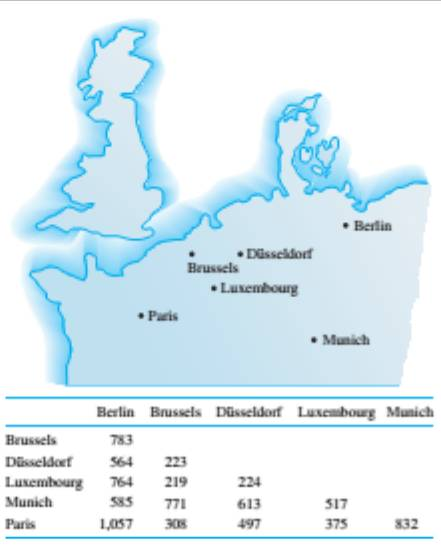Chapter 10.1, Problem 42ES### Discrete Mathematics With Applicat...

5th Edition
EPP + 1 other
ISBN: 9781337694193

#### Solutions

Chapter
Section### Discrete Mathematics With Applicat...

5th Edition
EPP + 1 other
ISBN: 9781337694193
Textbook Problem
18 views

# A traveler in Europe wants to visit each of the cities shown on the map exactly once, starting and ending in Brussels. The distance (in kilometer) between each pair of cities is given in the table. Find a Hamiltonian circuit that minimizes the total distance traveled. (Use the map to narrow the possible circuit down to just a few. Then use the table to find the total distance for each of those.)To determine

Find a Hamiltonian circuit that minimizes the total distance travelled. (Use the map to narrow the possible circuits down to just a few. Then use the table to find the total distance for each of those.)

Explanation

Given information:

A traveller in Europe wants to visit each of the cities shown on the map exactly once, starting and ending in Brussels. The distance (in kilometres) between each pair of cities is given in the table.

Calculation:

Let us first represent the given map by a graph, where each vertex is one of the cities in the map. We draw an edge between every pair of vertices and label the edge with the distance between the two cities.

Let us next determine all Hamilton circuits starting and ending in Brussels and the corresponding distances ( A = Brussels, B = Berlin, D = Düsseldorf, L = Luxembourg, M = Munich, P = Paris).

List the Hamilton circuits that make sense only.

• It does not make sense to go from Brussels to Munich or from Munich to Brussels, as they lie on opposite sides of the map.
• It does not make sense to go from Paris to Berlin or from Berlin to Paris, as they lie on opposite sides of the map.
• It does not make sense to change in the opposite direction on the next move: move from left to right and then back to the left on the next turn; or move from right to left and then back to the right on the next turn.

Note: If abcde fa is a Hamilton circuit of minimized distance, then a f edcba is also a Hamilton circuit of minimized distance. Hamilton circuit                      DistanceABDLMPA                      564+783+224+517+832+308=3228ABDMLPA

### Still sussing out bartleby?

Check out a sample textbook solution.

See a sample solution

#### The Solution to Your Study Problems

Bartleby provides explanations to thousands of textbook problems written by our experts, many with advanced degrees!

Get Started